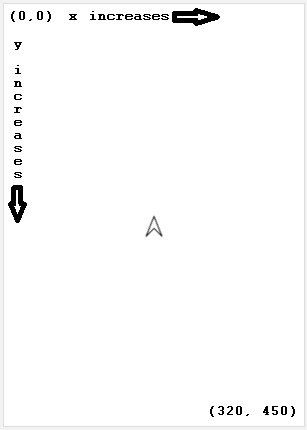# getY

Category:Turtle

## getY()

Category: Turtle

Gets the current y coordinate in pixels of the turtle.

The y coordinate is the distance from the turtle to the top of the screen.

### Examples

```var yLocation = getY();
console.log(yLocation);
moveTo(100, 100);
console.log(getY());
```

Top and to the Left Move a bit closer to the top left.

```// Move a bit closer to the top left.
var newX = getX() * 0.75;
var newY = getY() * 0.75;
moveTo(newX, newY);
```

Bounce the Turtle Have the turtle keep moving, but bounce off the walls so it stays on the screen.

```// Have the turtle keep moving, but bounce off the walls so it stays on the screen.
var speedX = 10;
var speedY = 10;
while (true) {
var newX = getX() + speedX;
var newY = getY() + speedY;
if (newX < 20) {
newX = 20;
speedX = - speedX;
} else if (newX > 300) {
newX = 300;
speedX = - speedX;
}
if (newY < 20) {
newY = 20;
speedY = - speedY;
} else if (newY > 460) {
newY = 460;
speedY = - speedY;
}
moveTo(newX, newY);
}
```

### Syntax

```getY();
```

### Parameters

getY() does not take any parameters.

### Returns

Returns a number representing the current y coordinate in pixels of the turtle within the app display.

### Tips

• The screen default size is 320 pixels wide and 450 pixels high, but you can move the turtle off the screen by exceeding those dimensions.
• The turtle can be moved off the screen so getX() can return a negative number if the turtle is off the screen to the left and getX() can return a number greater than 320 if the turtle is off the screen to the right.Found a bug in the documentation? Let us know at documentation@code.org

Found a bug in the documentation? Let us know at documentation@code.org